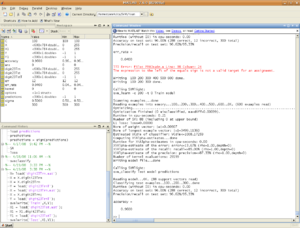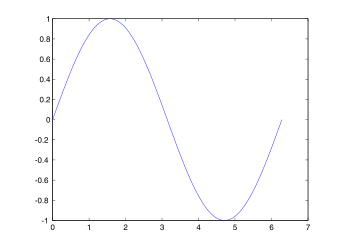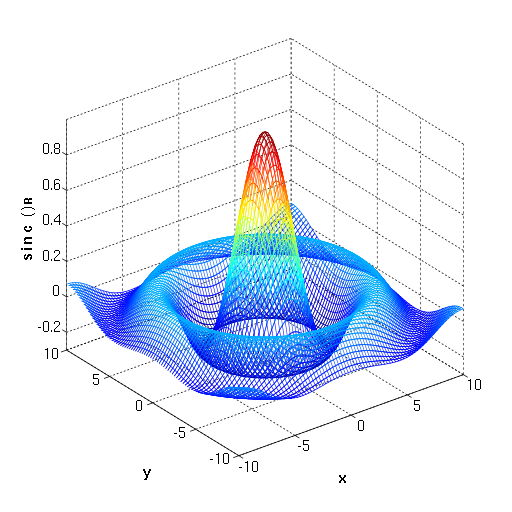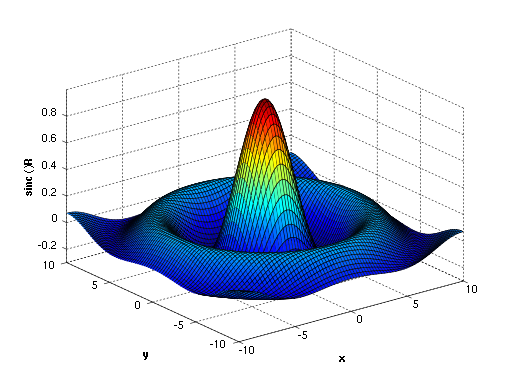# MATLAB

﻿
MATLAB
MATLABMATLAB R2011a screenshot
Developer(s) MathWorks
Stable release R2011b / September 1, 2011; 57 days ago
Written in C, Java
Operating system Cross-platform
Type Technical computing
Website MATLAB product page
Paradigm(s) multi-paradigm: imperative, procedural, object-oriented, array late 1970s Cleve Moler MathWorks dynamic, weak Cross-platform

MATLAB (matrix laboratory) is a numerical computing environment and fourth-generation programming language. Developed by MathWorks, MATLAB allows matrix manipulations, plotting of functions and data, implementation of algorithms, creation of user interfaces, and interfacing with programs written in other languages, including C, C++, Java, and Fortran.

Although MATLAB is intended primarily for numerical computing, an optional toolbox uses the MuPAD symbolic engine, allowing access to symbolic computing capabilities. An additional package, Simulink, adds graphical multi-domain simulation and Model-Based Design for dynamic and embedded systems.

In 2004, MATLAB had around one million users across industry and academia. MATLAB users come from various backgrounds of engineering, science, and economics. MATLAB is widely used in academic and research institutions as well as industrial enterprises and the Government of Argentina.

## History

Cleve Moler, the chairman of the computer-science department at the University of New Mexico, started developing MATLAB in the late 1970s. He designed it to give his students access to LINPACK and EISPACK without them having to learn Fortran. It soon spread to other universities and found a strong audience within the applied mathematics community. Jack Little, an engineer, was exposed to it during a visit Moler made to Stanford University in 1983. Recognizing its commercial potential, he joined with Moler and Steve Bangert. They rewrote MATLAB in C and founded MathWorks in 1984 to continue its development. These rewritten libraries were known as JACKPAC. In 2000, MATLAB was rewritten to use a newer set of libraries for matrix manipulation, LAPACK.

MATLAB was first adopted by researchers and practitioners in control engineering, Little's specialty, but quickly spread to many other domains. It is now also used in education, in particular the teaching of linear algebra and numerical analysis, and is popular amongst scientists involved in image processing. However, many researchers mostly from Computer Science background feel that MATLAB should be used only for mathematical analysis necessary in image processing and not for implementation of image processing software. Moreover, MATLAB should not be used to simulate computer architectures, systems software and computer networks unless while solving some numeric problem.[citation needed]

## Syntax

The MATLAB application is built around the MATLAB language. The simplest way to execute MATLAB code is to type it in the Command Window, which is one of the elements of the MATLAB Desktop. When code is entered in the Command Window, MATLAB can be used as an interactive mathematical shell. Sequences of commands can be saved in a text file, typically using the MATLAB Editor, as a script or encapsulated into a function, extending the commands available.

### Variables

Variables are defined as the assignment operator, `=`. MATLAB is a weakly dynamically typed programming language. It is a weakly typed language because types are implicitly converted. It is a dynamically typed language because variables can be assigned without declaring their type, except if they are to be treated as symbolic objects, and that their type can change. Values can come from constants, from computation involving values of other variables, or from the output of a function. For example:

```>> x = 17
x =
17
>> x = 'hat'
x =
hat
>> y = x + 0
y =
104        97       116
>> x = [3*4, pi/2]
x =
12.0000    1.5708
>> y = 3*sin(x)
y =
-1.6097    3.0000
```

### Vectors/matrices

As suggested by its name (a contraction of "Matrix Laboratory"), MATLAB can create and manipulate arrays of 1 (vectors), 2 (matrices), or more dimensions. In the MATLAB vernacular, a vector refers to a one dimensional (1×N or N×1) matrix, commonly referred to as an array in other programming languages. A matrix generally refers to a 2-dimensional array, i.e. an m×n array where m and n are greater than 1. Arrays with more than two dimensions are referred to as multidimensional arrays. Arrays are a fundamental type and many standard functions natively support array operations allowing work on arrays without explicit loops. Therefore the MATLAB language is also an example of array programming language.

A simple array is defined using the syntax: init`:`increment`:`terminator. For instance:

```>> array = 1:2:9
array =
1 3 5 7 9
```

defines a variable named `array` (or assigns a new value to an existing variable with the name `array`) which is an array consisting of the values 1, 3, 5, 7, and 9. That is, the array starts at 1 (the init value), increments with each step from the previous value by 2 (the increment value), and stops once it reaches (or to avoid exceeding) 9 (the terminator value).

```>> array = 1:3:9
array =
1 4 7
```

the increment value can actually be left out of this syntax (along with one of the colons), to use a default value of 1.

```>> ari = 1:5
ari =
1 2 3 4 5
```

assigns to the variable named `ari` an array with the values 1, 2, 3, 4, and 5, since the default value of 1 is used as the incrementer.

Indexing is one-based, which is the usual convention for matrices in mathematics, although not for some programming languages.

Matrices can be defined by separating the elements of a row with blank space or comma and using a semicolon to terminate each row. The list of elements should be surrounded by square brackets: []. Parentheses: () are used to access elements and subarrays (they are also used to denote a function argument list).

```>> A = [16 3 2 13; 5 10 11 8; 9 6 7 12; 4 15 14 1]
A =
16  3  2 13
5 10 11  8
9  6  7 12
4 15 14  1

>> A(2,3)
ans =
11
```

Sets of indices can be specified by expressions such as "2:4", which evaluates to [2, 3, 4]. For example, a submatrix taken from rows 2 through 4 and columns 3 through 4 can be written as:

```>> A(2:4,3:4)
ans =
11 8
7 12
14 1
```

A square identity matrix of size n can be generated using the function eye, and matrices of any size with zeros or ones can be generated with the functions zeros and ones, respectively.

```>> eye(3)
ans =
1 0 0
0 1 0
0 0 1
>> zeros(2,3)
ans =
0 0 0
0 0 0
>> ones(2,3)
ans =
1 1 1
1 1 1
```

Most MATLAB functions can accept matrices and will apply themselves to each element. For example, `mod(2*J,n)` will multiply every element in "J" by 2, and then reduce each element modulo "n". MATLAB does include standard "for" and "while" loops, but (as in other similar applications such as R), using the vectorized notation often produces code that is faster to execute. This code, excerpted from the function magic.m, creates a magic square M for odd values of n (MATLAB function meshgrid is used here to generate square matrices I and J containing 1:n).

```[J,I] = meshgrid(1:n);
A = mod(I+J-(n+3)/2,n);
B = mod(I+2*J-2,n);
M = n*A + B + 1;
```

### Semicolons

Unlike many other languages, where the semicolon is used to terminate commands, in MATLAB the semicolon serves to suppress the output of the line that it concludes (it serves a similar purpose in Mathematica).

### Graphics

Function plot can be used to produce a graph from two vectors x and y. The code:

```x = 0:pi/100:2*pi;
y = sin(x);
plot(x,y)
```

produces the following figure of the sine function:Three-dimensional graphics can be produced using the functions surf, plot3 or mesh.

 ```[X,Y] = meshgrid(-10:0.25:10,-10:0.25:10); f = sinc(sqrt((X/pi).^2+(Y/pi).^2)); mesh(X,Y,f); axis([-10 10 -10 10 -0.3 1]) xlabel('{\bfx}') ylabel('{\bfy}') zlabel('{\bfsinc} ({\bfR})') hidden off ``` ```[X,Y] = meshgrid(-10:0.25:10,-10:0.25:10); f = sinc(sqrt((X/pi).^2+(Y/pi).^2)); surf(X,Y,f); axis([-10 10 -10 10 -0.3 1]) xlabel('{\bfx}') ylabel('{\bfy}') zlabel('{\bfsinc} ({\bfR})') ``` This code produces a wireframe 3D plot of the two-dimensional unnormalized sinc function: This code produces a surface 3D plot of the two-dimensional unnormalized sinc function:### Structures

MATLAB supports structure data types. Since all variables in MATLAB are arrays, a more adequate name is "structure array", where each element of the array has the same field names. In addition, MATLAB supports dynamic field names (field look-ups by name, field manipulations etc). Unfortunately, MATLAB JIT does not support MATLAB structures, therefore just a simple bundling of various variables into a structure will come at a cost.[citation needed]

### Function handles

MATLAB supports elements of lambda-calculus by introducing function handles, or function references, which are implemented either in .m files or anonymous/nested functions.

### Secondary programming

MATLAB also carries secondary programming which incorporates the MATLAB standard code into a more user friendly way to represent a function or system.

### Classes

Although MATLAB supports classes, the syntax and calling conventions are significantly different from other languages. MATLAB supports value classes and reference classes, depending if the class has handle as super-class (for reference classes) or not (for value classes).

Depending if a class is declared as value or reference, method call behavior is different. For example, a call to a method

```object.method();
```

can alter any member of object only if object is an instance of a reference class.

## Object-oriented programming

MATLAB's support for object-oriented programming includes classes, inheritance, virtual dispatch, packages, pass-by-value semantics, and pass-by-reference semantics.

```classdef hello
methods
function doit(this)
disp('Hello!')
end
end
end
```

When put into a file named hello.m, this can be executed with the following commands:

```>> x = hello;
>> x.doit;
Hello!
```

## Interfacing with other languages

MATLAB can call functions and subroutines written in the C programming language or Fortran. A wrapper function is created allowing MATLAB data types to be passed and returned. The dynamically loadable object files created by compiling such functions are termed "MEX-files" (for MATLAB executable).

Libraries written in Java, ActiveX or .NET can be directly called from MATLAB and many MATLAB libraries (for example XML or SQL support) are implemented as wrappers around Java or ActiveX libraries. Calling MATLAB from Java is more complicated, but can be done with MATLAB extension, which is sold separately by MathWorks, or using an undocumented mechanism called JMI (Java-to-Matlab Interface), which should not be confused with the unrelated Java Metadata Interface that is also called JMI.

As alternatives to the MuPAD based Symbolic Math Toolbox available from MathWorks, MATLAB can be connected to Maple or Mathematica.

Libraries also exist to import and export MathML.

MATLAB is a proprietary product of MathWorks, so users are subject to vendor lock-in. Although MATLAB Builder can deploy MATLAB functions as library files which can be used with .NET or Java application building environment, future development will still be tied to the MATLAB language.

### Prices

Each toolbox is purchased separately.

If an evaluation licence is requested, the MathWorks sales department requires detailed information about the project for which MATLAB is to be evaluated. Overall the process of acquiring a licence is expensive in terms of money and time. If at all granted (which happens often), the evaluation licence is valid for two weeks. Student version of MATLAB are sold at bookstores and what the professors encourages them to buy, though standard version is what the universities and colleges installed in labs.

## Alternatives

MATLAB has a number of competitors. Commercial competitors include Mathematica, Maple, IDL by ITT Visual Information Solutions and Metlynx.

There are also free open source alternatives to MATLAB, in particular GNU Octave, FreeMat, and Scilab which are intended to be mostly compatible with the MATLAB language (but not the MATLAB desktop environment).

Among other languages that treat arrays as basic entities (array programming languages) are APL and J, Fortran 95 and 2003, as well as the statistical language S (the main implementations of S are S-PLUS and the popular open source language R).

There are also several libraries to add similar functionality to existing languages, such as Itpp for C++, Perl Data Language for Perl, ScalaLab for Scala and SciPy together with NumPy and Matplotlib for Python.

## Release history

Version Release name Year Release Date Notes
MATLAB 1.0 1984
MATLAB 2 1986
MATLAB 3 1987
MATLAB 3.5 1990 Ran on MS-DOS but required at least a 386 processor. Version 3.5m required math coprocessor
MATLAB 4 1992
MATLAB 4.2c R7 1994 Ran on Windows 3.1. Required a math coprocessor
MATLAB 5.0 R8 1996 December, 1996
MATLAB 5.1 R9 1997 May, 1997
MATLAB 5.1.1 R9.1
MATLAB 5.2 R10 1998 March, 1998
MATLAB 5.2.1 R10.1
MATLAB 5.3 R11 1999 January, 1999
MATLAB 5.3.1 R11.1 November, 1999
MATLAB 6.0 R12 2000 November, 2000
MATLAB 6.1 R12.1 2001 June, 2001
MATLAB 6.5 R13 2002 July, 2002
MATLAB 6.5.1 R13SP1 2003
MATLAB 6.5.2 R13SP2
MATLAB 7 R14 2004 June, 2004
MATLAB 7.0.1 R14SP1 October, 2004
MATLAB 7.0.4 R14SP2 2005 March 7, 2005
MATLAB 7.1 R14SP3 September 1, 2005
MATLAB 7.2 R2006a 2006 March 1, 2006
MATLAB 7.3 R2006b September 1, 2006 HDF5-based MAT-file support
MATLAB 7.4 R2007a 2007 March 1, 2007
MATLAB 7.5 R2007b September 1, 2007 Last release for Windows 2000 and PowerPC Mac. License Server support for Windows Vista
MATLAB 7.6 R2008a 2008 March 1, 2008
MATLAB 7.7 R2008b October 9, 2008
MATLAB 7.8 R2009a 2009 March 6, 2009 First release for 32-bit & 64-bit Windows 7.
MATLAB 7.9 R2009b September 4, 2009 First release for Intel 64-bit Mac, and last for Solaris SPARC.
MATLAB 7.9.1 R2009bSP1 2010 April 1, 2010
MATLAB 7.10 R2010a March 5, 2010 Last release for Intel 32-bit Mac.
MATLAB 7.11 R2010b September 3, 2010
MATLAB 7.11.1 R2010bSP1 2011 March 17, 2011
MATLAB 7.12 R2011a April 8, 2011
MATLAB 7.13 R2011b September 1, 2011

## File Extensions

### Native

.fig
MATLAB Figure
.m
MATLAB function, script, or class
.mat
MATLAB binary file for storing variables
.mex...
MATLAB executable (platform specific, e.g. ".mexmac" for the Mac, ".mexglx" for Linux, etc.)
.p
MATLAB content-obscured .m file (result of pcode() )

### Third-party

.jkt
GPU Cache file generated by Jacket for MATLAB (AccelerEyes)
.mum
MATLAB CAPE-OPEN Unit Operation Model File (AmsterCHEM)

## Easter Eggs

Several easter eggs exist in the software. These include hidden pictures, and random in-jokes.

Wikimedia Foundation. 2010.

### Look at other dictionaries:

• MATLAB — Логотип Тип Программы математического моделирования Разработчик …   Википедия

• Matlab — R2008a Screenshot …   Deutsch Wikipedia

• MATLAB — Desarrollador MathWorks www.mathworks.com/products/matlab …   Wikipedia Español

• MATLAB — Développeur MathWorks Dernière version …   Wikipédia en Français

• Matlab — Développeur The MathWorks Dernière version …   Wikipédia en Français

• MATLAB — Entwickler: The MathWorks Aktuelle Version: 7.8 (R2009a) (6. März 2009) Betriebssystem: Linux, Unix, Windows, Mac OS X …   Deutsch Wikipedia

• MATLAB — es la abreviatura de Matrix Laboratory (laboratorio de matrices). Es un programa de matemáticas creado por The MathWorks en 1984. Está disponible para las plataformas Unix, Windows y MAC. Se pueden ampliar sus capacidades con Toolboxes, algunas… …   Enciclopedia Universal

• MatLab — …   Википедия

• Matlab — …   Википедия

• matlab — Inglish (Indian English) Dictionary What I mean is (from Hindi) …   English dialects glossary